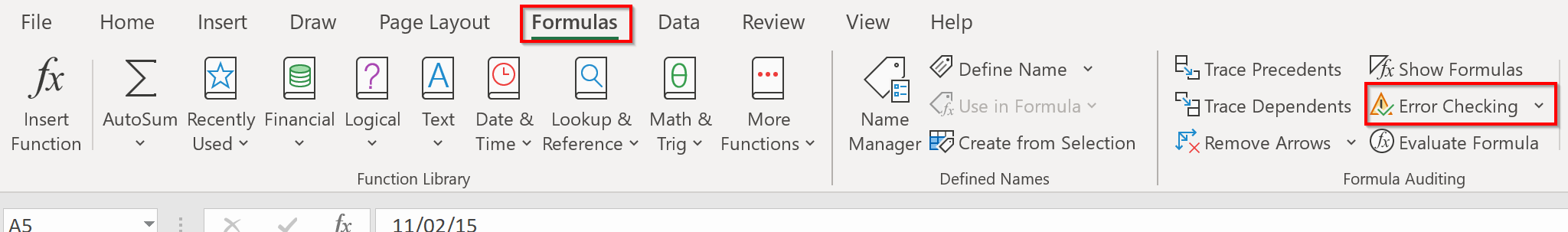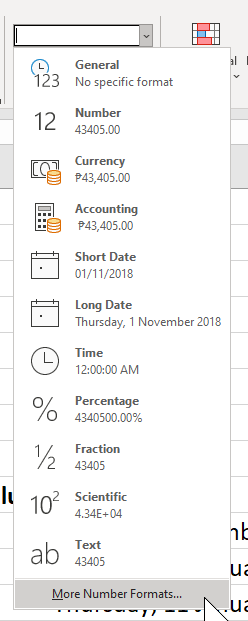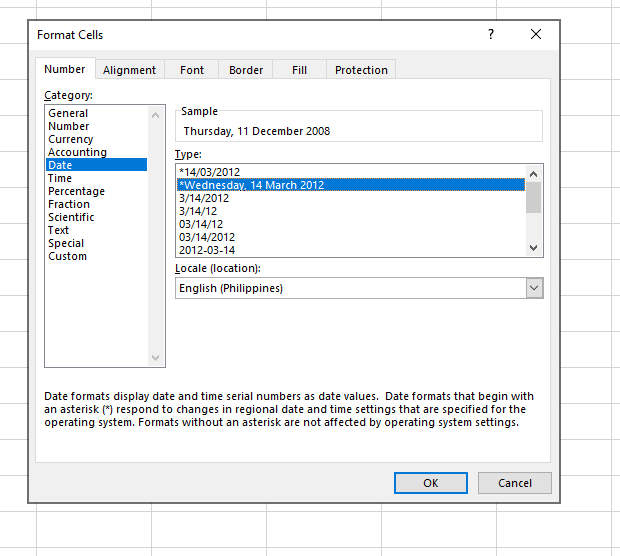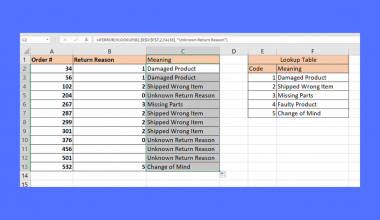# How to Convert a Date Stored as Text to a Date Value in Excel

The DATEVALUE function and error checking tool is useful if you need to convert dates stored as text to date values in Excel.

We can use the DATEVALUE function to get the actual date from the text in a date format that Microsoft Excel recognizes. This is useful when the data you have is not inserted as a date value.

The rules for using the `DATEVALUE` function in Excel are as follows:

• The function requires a date text as its sole argument.
• The function then outputs the serial number of that particular date.

The advantage of outputting a serial number is that Excel can later use that value to convert into any chosen format you want to.

Let’s take a look at a quick example where we can use the `DATEVALUE` function.

You have a dataset of vehicle registration data. The date column has been difficult to work with since each registration office uses a different format in their own Excel documents.We can use the `DATEVALUE` function to convert every date into a standard format later. This is done by getting the serial number of each date. The serial number is simply the number of days since January 1st, 1900.

For example, the date December 12, 2005, has a serial number of 38698. This simply means that the date is 38,698 days after January 1st, 1900.

Another way we can convert text to dates is through error checking.

A common error when importing data is that the original text only specified the last two digits of the year. Common formats such as “01/01/20” or “01-Jan-20” can cause some confusion later on. Does the date mean the 1st of January in 1920 or 2020?

Excel has a built-in error checking feature that looks for these types of errors. Once these errors are caught, Excel converts them into a valid Date.

Now that we have an idea of when to use these functions, let’s look at some real examples of them in action.

## A Real Example of Converting a Date Stored as Text to a Date Value

Let’s take a look at sample conversion made using the `DATEVALUE` function.

In the example below, we have multiple text values that follow different date formats. Column B uses the `DATEVALUE` function to convert each of these formats into their respective serial numbers.To get the values in Column B, we just need to use the following formula:

`=DATEVALUE(A10)`

In this next example, we used the Error Checking tool to easily convert dates with ambiguous years into a proper date format.You can make your own copy of the spreadsheet above using the link attached below.

If you’re ready to test these functions yourself in Excel, follow our guide in the next section!

## How to Convert a Date Stored as Text to a Date Value in Excel

This section will show you each step needed to convert dates stored as text to their date values. You’ll learn how we can use the `DATEVALUE` function and the Error Checking tool to accomplish this.

Follow these steps to start using the Error Checking function:

1. If any of your dates has a green marker on the top-left corner of the cell, you might have to check for errors. In the table below, each of these dates has only the last two digits of their year.This would be fine if Excel stored its serial number, but you have noticed that it was imported as text values.
2. In the Formulas tab, click on the Error Checking option.3. A dialog box will appear that will help you handle each ambiguous date. Excel makes it easy to convert a year written as “20” to mean either 1920 or 2020.Excel will let you know once all errors have been dealt with.
4. After using the Error Checking tool, your dates should now be specific and can now be treated as a valid date.These next few steps will now show you how you can use the `DATEVALUE` function in Excel to convert your text to dates:

1. Write down “=DATEVALUE(“ into the formula bar and indicate the target cell to convert to a serial number. In the example below, we converted the date in A10 to the serial number 39793.2. Fill down the rest of the column by dragging the first instance of the `DATEVALUE` formula.3. We can now convert these serial numbers into any date format we want. Simply select the range and look for a format you want in the dropdown menu.You can click on the More Number Formats… option to find even more date formats to choose from.4. Click OK. You should now have a date value you can read that is uniform for the entire column.## Frequently Asked Questions (FAQ)

1. What happens if the serial value of a year is not indicated?
Excel assumes that dates without a year take place in the current year. For example, if you try to get the date value of “01-JAN” you will get a date value equivalent to the 1st of January of the current year.That’s all you need to remember to start using the `DATEVALUE` function in Microsoft Excel. This step-by-step guide should be all you need to convert a date stored as text to a date value.

The `DATEVALUE` and Error Checking functions are just a few examples of helpful functions to deal with cell data in Microsoft Excel. With so many other Excel functions out there, you can surely find one that suits your needs.

Are you interested in learning more about what Excel can do? Stay notified of new guides like this by subscribing to our newsletter!### Get emails from us about Excel.

Our goal this year is to create lots of rich, bite-sized tutorials for Excel users like you. If you liked this one, you'd love what we are working on! Readers receive ✨ early access ✨ to new content.

##### You May Also Like## How to Fix Hyperlink to Website Not Working in Excel

This guide will discuss how to fix hyperlink to website not working in Excel. A hyperlink is a…## How to Create a Gauge Chart in Excel

The gauge chart in Excel is useful when you want to create an eye-catching visualization for a certain…## How to Remove Drop-down List in Excel

This guide will explain how you can remove a drop-down list in Excel. Since drop-down lists are a…## How to Apply Different Types of Conditional Formatting in Excel

This guide will discuss how to apply different types of conditional formatting in Excel. Conditional formatting in Excel…